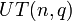# Conjugacy class sizes of unitriangular matrix group of fixed degree over a finite field are all powers of field size and number of occurrences of each is polynomial function of field size

## Statement

Denote by$UT(n,q)$ the unitriangular matrix group of degree$n$ over a finite field of size$q$, where$q$ is a prime power.

Then, the following is true about the conjugacy class size statistics of$UT(n,q)$:

• All the conjugacy class sizes are powers of$q$.
• For any nonnegative integer$d$, there exists a univariate polynomial$g_{n,d}$ with integer coefficients (dependent on$n$ and$d$ but independent of$q$) such that the number of conjugacy classes in$UT(n,q)$ of size$q^d$ equals$g_{n,d}(q)$. Further, we have, based on the fact that the sum of all conjugacy class sizes must equal the order of the group, that the following is true as a polynomial identity in$q$:$\sum_{d=0}^\infty q^{d}g_{n,d}(q) = q^{n(n-1)/2}$

Note that the summation is actually finite, because all but finitely many of the$g_{n,d}$ are zero polynomials.